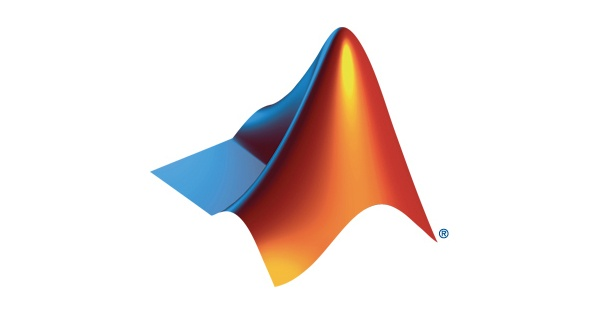SOFTWARE CATEGORIES

# MATLAB Software Features, Pricing, Alternatives & Review 2022No items found.

MATLABSOFTWARE REVIEW
MATLABWhat is MATLABMATLAB BenefitsMATLAB FeaturesMATLAB PricingMATLAB Software Alternatives## What is MATLAB

MATLAB is a high-performance language for technical computing. It integrates computation, visualization, and programming in an easy-to-use environment where problems and solutions are expressed in familiar mathematical notation.

MATLAB is an interactive system whose basic data element is an array that does not require dimensioning. This allows you to solve many technical computing problems, especially those with matrix and vector formulations, in a fraction of the time it would take to write a program in a scalar noninteractive language such as C or Fortran.

MATLAB is Artificial Intelligence Software that can grow your business. More details soon!

WHAT IS THE POTENTIAL VALUE OF THIS SOFTWARE

## MATLAB Benefits

No items found.
WHICH FEATURES STAND OUT THE MOST OF THIS SOFTWARE

## MATLAB Features

WHAT ARE THE PRICING PLANS of this software

No items found.